1
0
0理解随机森林：基于Python的实现和解释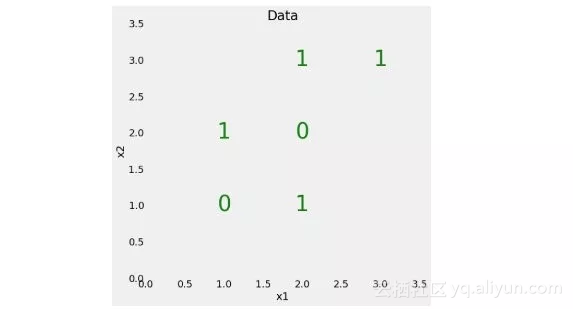from sklearn.tree import DecisionTreeClassifier
# Make a decision tree and train

tree = DecisionTreeClassifier(random_state=RSEED)
tree.fit(X, y)

print(f'Model Accuracy: {tree.score(X, y)}')

Model Accuracy: 1.0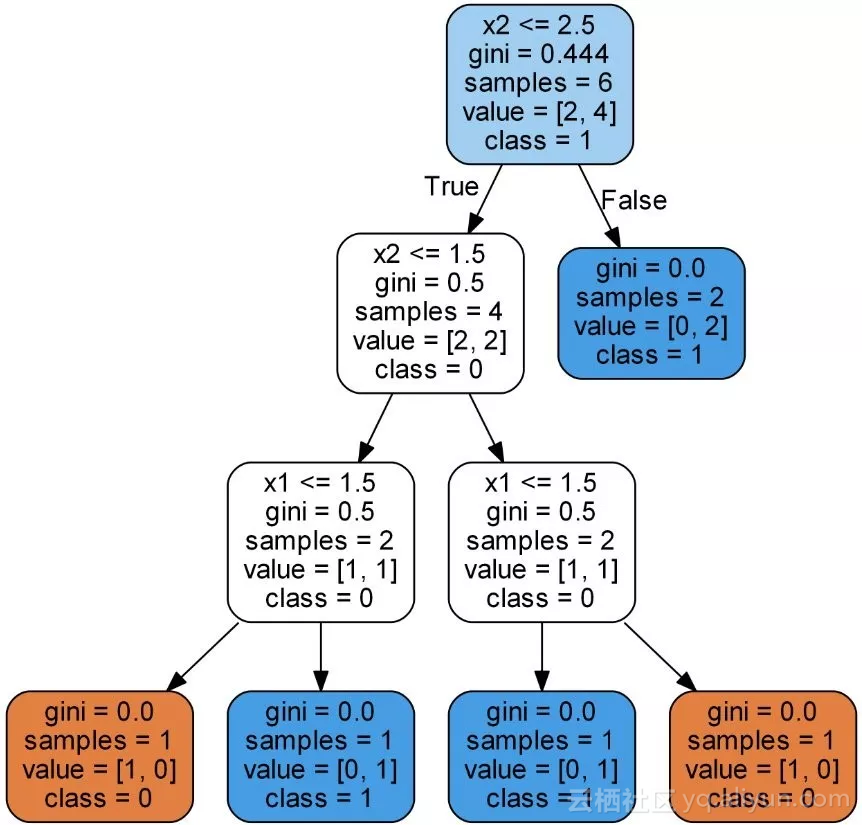gini：节点的基尼不纯度。当沿着树向下移动时，平均加权的基尼不纯度必须降低。

samples：节点中观察的数量。

value：每一类别中样本的数量。比如，顶部节点中有 2 个样本属于类别 0，有 4 个样本属于类别 1。

class：节点中大多数点的类别（持平时默认为 0）。在叶节点中，这是该节点中所有样本的预测结果。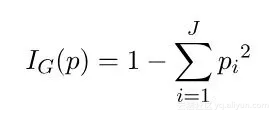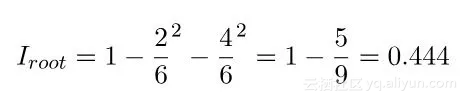1.随机采样数据点

2.基于特征的子集分割节点

from sklearn.ensemble import RandomForestClassifier

# Create the model with 100 trees

model = RandomForestClassifier(n_estimators=100,
random_state=RSEED,
max_features = 'sqrt',
n_jobs=-1, verbose = 1)

# Fit on training data

model.fit(train, train_labels)

rf_predictions = model.predict(test) rf_probs = model.predict_proba(test)[:, 1]

from sklearn.metrics import roc_auc_score

# Calculate roc auc

roc_value = roc_auc_score(test_labels, rf_probs)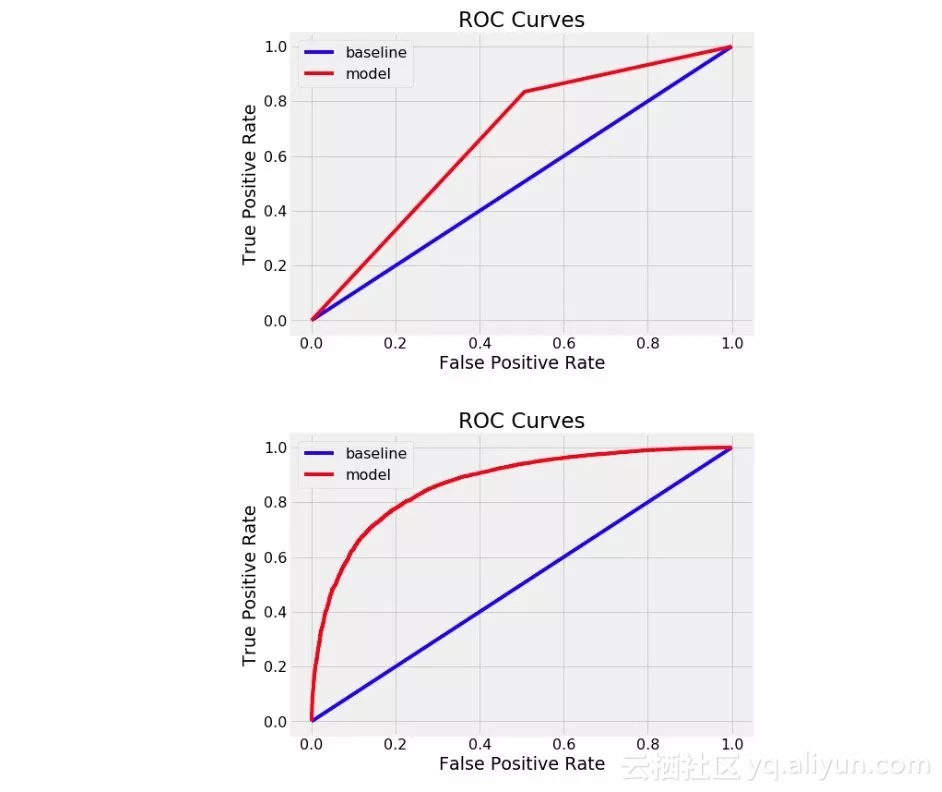import pandas as pd

fi = pd.DataFrame({'feature': list(train.columns),
'importance': model.feature*importances*}).
sort_values('importance', ascending = False)

feature importance
tDIFFWALKt0.036200
tQLACTLM2t0.030694
tEMPLOY1t 0.024156
tDIFFALONt0.022699
tUSEEQUIPt0.016922
tDECIDEt 0.016271
t_LMTSCL1t0.013424
tINCOME2t 0.011929
tCHCCOPD1t0.011506
t_BMI5t 0.011497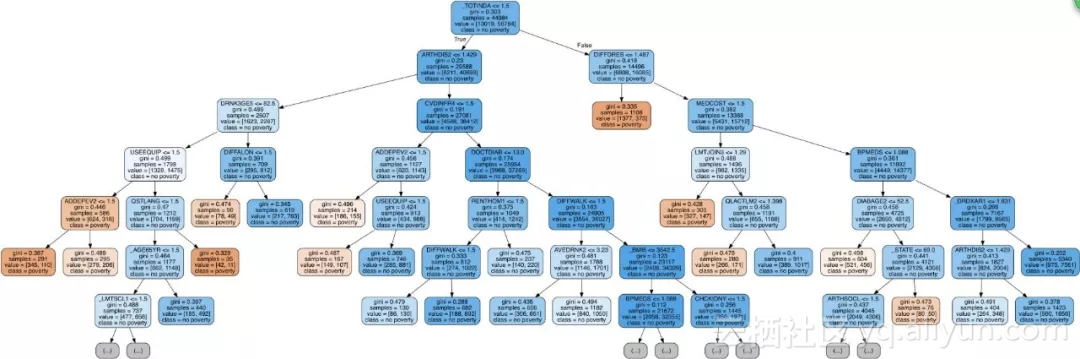bootstrapping：可重复地采样随机观察集。随机森林用于训练每个决策树的方法。

+ 关注